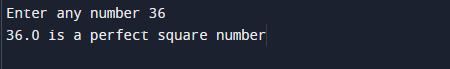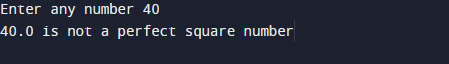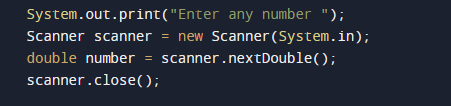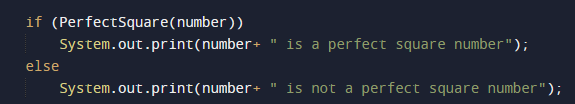# Java Program to check if a given number is perfect square

In this tutorial you will learn about the Java Program to check if a given number is perfect square and its application with practical example.

In this tutorial, we will learn to create a Java Program to check if a given number is perfect square using Java programming.

## Prerequisites

Before starting with this tutorial we assume that you are best aware of the following Java programming topics:

• Java Operators.
• Basic Input and Output function in Java.
• Class and Object in Java.
• Basic Java programming.
• If-else statements in Java.
• For loop in Java.
• User Define functions

## What is perfect square.

A number that can be expressed as the square of a number .in other word it is the product of number with itself.

9 is a perfect square number, since it equals 3² =3*3=9.

## Java Program to check if a given number is perfect square.

In this program we would find the Perfect Square  of a given number .First of all we take a value form user and find the Perfect Square of a number.Let have a look at the code.

## Output

Perfect numberNot  a Perfect NumberIn the above program, we have first declared and initialized a set variables required in the program.

• number= it will hold entered number.
• sqrt=  it will hold the result.

After declaring variables we take a value from user and find Perfect Square  of a number..after taking value from user add to variable  number and pass the number to function PerfectSquare(number) where we check condition for prefect square as shown in the image below.this function calculate perfect square using math.sqrt() method to find perfect square of  a number .here value of a given number is true then if statement will executed if not else statement will be  executed

In this tutorial we have learn about the Java Program to check if a given number is perfect square and its application with practical example. I hope you will like this tutorial.